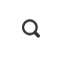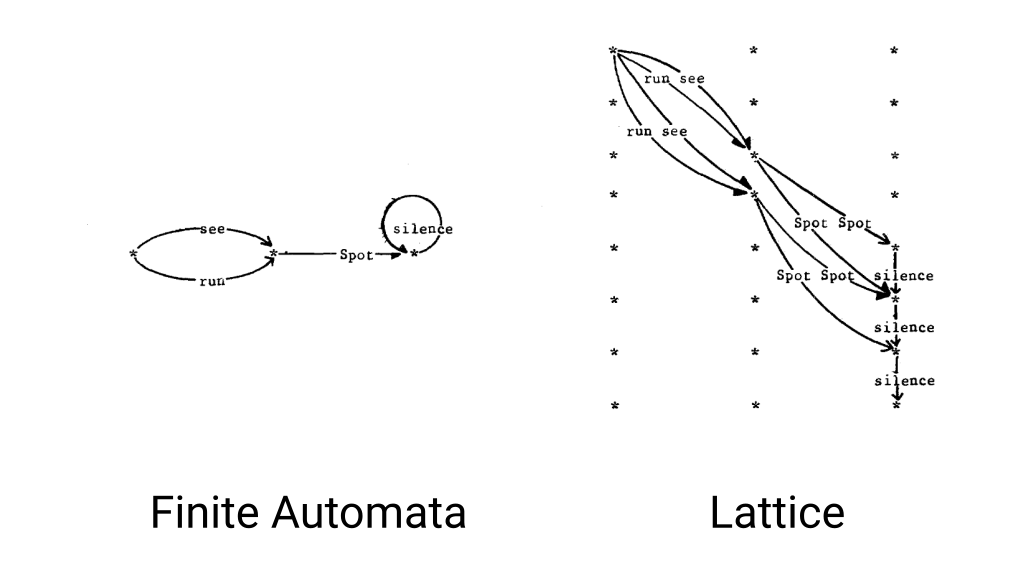• Costs: Are Log Negative Probability, so a higher cost means lower probability.
 • Frame: Each 10ms of audio that using MFCC turned into a fixed size vector called a frame.
 • Beam: Cutoff would be `Best Cost`–`Beam` (Around 10 to 16)
 • Cutoff: The maximum cost that all cost higher than this value will not be processed and removed.
 • Epsilon: The zero label in `FST` are called ``
 • Lattices: Are the same as FSTs, instead each token keeps in a framed based array called`frame_toks`. In This way the distance in time between each token will be perceived too.
 • Rescoring: A language model scoring system that applied after final state to improve final result by using stronger LM model than `n-gram`.
 • HCLG(FST): The main FST used in the decoding. The iLabel in this FST is TransitionIDs.
 • Model(MDL): A model that used to convert sound into acoustic cost and TransitionIDs.
 • TransitionIDs: A number that contain information about state and corresponding PDF id.
 • Emiting States: States that have pdfs associated with them and emit phoneme. In other word states that have their `ilabel` is not zero
 • Bakis Model: Is a HMM that state transitions proceed from left to right. In a Bakis HMM, no transitions go from a higher-numbered state to a lower-numbered state.
 • Max Active: Uses to calculate cutoff to determince maximum number of tokens that will be processed inside emitting process.
 • Graph Cost: is a sum of the LM cost, the (weighted) transition probabilities, and any pronunciation cost.
 • Acoustic Cost: Cost that is got from the decodable object.
 • Acoustic Scale: A floating number that multiply in all Log Likelihood (inside the decodable object).Fig. 1. Demonstration of Finite State Automata vs Lattices, Courtesy of Peter F. Brown

Recent Posts